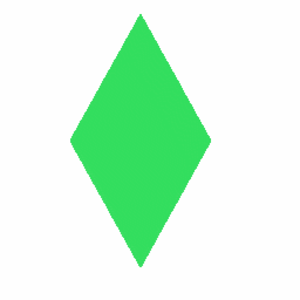# A Rhombus Has How Many Sides?

A rhombus has four sides. It is a quadrilateral with all four sides equalling the same length. The rhombus is often called a diamond, named after the diamond suit in playing cards, or can be referred to as a lozenge. However, a lozenge usually refers to a rhombus with a 45 degree angle. Every rhombus is also a parallelogram, this means that it has two pairs of parallel sides. A rhombus with right angles is referred to as a square. Most modern mathematicians include squares within a definition of a rhombus but the original definition by Euclid does not. The word ‘rhombus’ comes from the Ancient Greek term ‘rhombos’ meaning ‘spinning top’.

A rhombus has a number of properties. Every rhombus has two diagonals that connect pairs of opposite vertices as well as two pairs of parallel sides. It is possible to prove that the rhombus is symmetric across each diagonal by using congruent triangles. The opposite angles of a rhombus have equal measure and the two diagonals are perpendicular. This means that a rhombus is an orthodiagonal quadrilateral. The diagonals of a rhombus must also bisect opposite angles.  Every rhombus is a parallelogram but not every parallelogram is a rhombus. However, any parallelogram with perpendicular diagonals is. As a general rule, if a parallelogram has perpendicular diagonals, one of which is a line of symmetry, it is known as a kite. Every rhombus is a kite while any quadrilateral that is both a parallelogram and a kite is a rhombus.

When used in mathematics a dual polygon of a rhombus is a rectangle. Three dimensional analogues of a rhombus can include both a bipyramid and a bicone. One of the five types of 2D lattice is the rhombus lattice which can also be referred to as the centered rectangular lattice. Identical rhombuses in mathematics can tile the 2D plane in three different ways. For the 60 degree rhombus this includes the Rhombille tiling.
thanked the writer.
Rhombus is an equilateral quadrilateral. It has four sides. Kite is an example of a rhombus.It has four sides its like a kite or a slightly shifted square.
thanked the writer.
I think that a rhombus has about 4 sides. I may be wrong but the geometry text book says 4
thanked the writer.
A rhombus is a quadrilateral. A quadrilateral has 4 sides. Thus, a rhombus has 4 sides.
thanked the writer.
A rombus has four sides with opposite sides being parallel and the same length. Also opposite angels also are the same.
thanked the writer.
Four sides
thanked the writer.